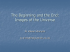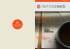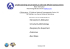# Lorenz system II - Harvard Mathematics Department

## Transcription

Lorenz system II - Harvard Mathematics Department
```THE LORENZ SYSTEM II
Math118, O. Knill
ABSTRACT. This is a continuation of the discussion about the Lorenz system and especially on the r dependence of the attractor.
OVERVIEW OVER BIFURCATIONS. We fix the parameter σ = 10, b = 8/3.
For 0 < r < 1, the origin is the only equilibrium point and all
points attracted to this point (you can find a proof in the book. At
r = 1, a pitchfork bifurcation takes place. The origin becomes
unstable and two stable equilibrium points appear. The picture
shows the case r = 1.5.
At the parameter r = 28, one observes the Lorenz attractor.
Between r = 0.99524 and r = 100.795, one observes an infinite series of period doubling bifurcations of stable periodic points (one
has to start with the larger value and decrease r). These bifurcations are analogue to the Feigenbaum scenario. The picture shows
the parameter r = 100.
For 1 < r < 13.925, the unstable manifold of the origin connects
to the equilibrium points. The picture shows r = 10.
Here we see the previous stable periodic cycle doubled. The parameter is r = 99.7. The period doubling scenario leads to the
same Feigenbaum constant as one can see in the one dimensional
logistic map family.
For r = r0 = 13.926, the unstable manifold becomes double
asymptotic to the origin.
At the parameter r0 , two unstable cycles appear. For 13.926 <
r < 24.06, these cycles come closer to the fixed points C ± . The
picture shows the parameter r = 20.
RETURN MAP. A good Poincare map is part of the
subplane z = r − 1. This plane contains the equilibrium point C ± . These points are fixed points of the
return map.
HISTORICAL. Lorenz carried out numerical investigations following work of Saltzman (1962). The Lorenz
equations can be found in virtually all books on dynamics. We consulted:
At the parameter r = r1 = 24.74 = 470/19, the unstable cycles collide with the stable equilibrium points and render them
unstable. This is called a subcritical Hopf bifurcation.
• C. Sparrow, ”The Lorenz equations: Bifurcations, chaos and strange attractors, Springer Verlag, 1982
• Strogatz, ”Nonlinear dynamics and Chaos”, Addison Wesley, 1994
• Dynamical systems X, Encyclopadia of Mathematics vol 66, Springer 1988
• Dennis Gulick, Encounters With chaos, Mc Graw-Hill, 1992
• Clark Robinson, Dynamical systems, Stability, Symbolic Dynamics and Chaos, CRC priss, 1995
```

### Pepper Choplin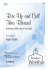### Document 6498116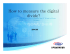### dr. edward norton loreNZ - University of Wisconsin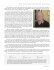### Devon Lorenz - Luginbuel Funeral Home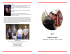### THREE DIMENSIONAL SYSTEMS Lecture 6: The Lorenz Equations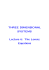exponents for an n-dimensional system, defined as follows... Consider the evolution of an infinitesimal sphere (in phase space) of perturbed initial conditions. During its evolution the sphere beco...

### State of the system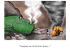### Professor Debraj Ray 19 West 4th Street, Room 608 email: , homepage: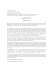### Tutorial of the STRUCTURE software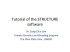### Document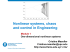### Vadim S. Anishchenko. - Dynamics, Bifurcations, and Strange### (June 2012) - D. INOUE (NICT Japan).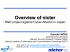### 1 SYLLABUS Course: General Chemistry II: CHEM-1100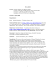### Lecture 1 - Biostatistics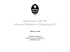### THE BUTTERFLY EFFECT### Delta Inverter ATC Settings for Delta VFD-V series inverter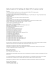### Geomorphology Uniformitarianism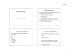### How a Sample PL/SQL Block Looks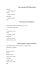### Le Châtelier`s Principle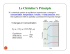### Capital-Induced Labor Migration in a Spatial Solow Model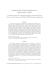### The Beginning and the End: Images of the Universe